# What is Taylor Series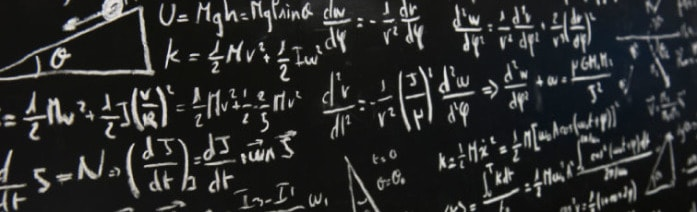Suppose you need to calculate $\sin{138}$ and don’t have a calculator at hand. How’d you do that? The way out is to approximate your function with something more convenient to work with, for example, polynomials: $x, x^2, x^3$ and so on. In this section, we’re going to discuss Taylor series which is an expansion of function into infinite sum of power functions. The series is called in honor of English mathematician Brook Taylor, though it was known before Taylor’s works. Taylor series is applied for approximation of function by polynomials. Such approach allows to replace initial more or less complicated function with the sum of simpler ones. Let’s get started.

Suppose we want to approximate some function $f(x)$ at the vicinity of some point $x_0$.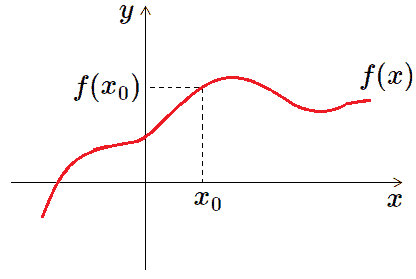We need a function which will resemble behavior of the given function $f(x)$ at some neighborhood of the point $x_0$. Surely, at least we can take a constant: $f_0=f(x_0)$ which equals $f(x)$ at the point $x_0$.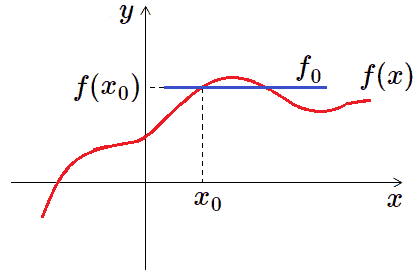Thus, $f_{approx}=f(x_0)$  is a horizontal straight line as you can see. But what about slope of $f(x)$ (in other words, the first derivative) at the point $x_0$? Obviously, $f_{approx}(x)$ doesn’t approximate that because $f’_{approx}=0$ at any point. Initial function $f(x)$ is a curve, while our approximation $f_{approx}$ is just a horizontal line. Not a great approximation indeed. By the way, it’s called zero degree approximation because $f_0$ is a zero degree polynomial function. To construct function which will approximate $f(x)$ along with its first derivative at the point $x_0$ we should add something to $f_0$. And this addend should be chosen so that it’ll be equal to zero at the point $x_0$. Let’s add the following term:

$$f_1(x)=(x-x_0)f’(x_0)$$

Factor $(x-x0)$ provides that our new updated expression holds the value of the initial function at the point $x_0$:

$$f_1(x_0)=(x_0-x_0)f’(x_0)=0$$

Video version of this tutorial is available on our youtube channel:

Thus, we obtain:

$$f_{approx}(x)=f_0+f_1=f(x_0)+(x-x_0)f’(x_0)$$

This is called the first degree approximation because $f_0+f_1$ is the first degree polynomial. As we can see, now the following holds for approximation function $f_{approx}$:

$$f_{approx}(x_0)=f(x_0)$$

$$f’_{approx}(x_0)=f’(x_0)$$

$$f’’_{approx}(x)=0$$

This time we’ve also obtained a straight line, but its slope at the point x_0 is the same as the slope of initial function $f(x)$.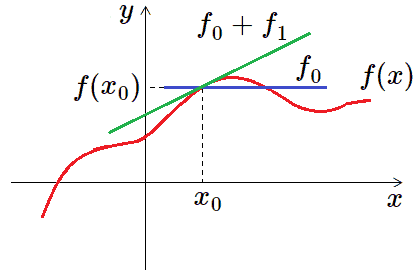But still our approximation is not very good. Let’s continue. We want now to add something to $f_{approx}$ so that it could approximate the second derivative of $f(x)$. Consider the following term:

$$f_2(x)=\frac{(x-x_0)^2}{2}f’’(x_0)$$

As you can see, along with $(x-x_0)^2$ there appears factor $\frac{1}{2}$. It’s because when we differentiate square, appears $2$ and so $\frac{1}{2}\cdot 2=1$ and we get rid of these integers.

$$f_{approx}(x)= f_0+f_1+f_2=f(x_0)+\frac{(x-x_0)^2}{2}f’’(x_0)$$

We’ve obtained a parabolic (quadratic) function, and that’s why it’s called the second degree approximation. As you may notice, each time we add terms the following condition hold: every new term turns into zero at $x_0$ and also gives zero at derivatives except the highest one it approximates. Particularly, $f_2$ approximates the second derivative. So $f_2$ turns into zero at the point $x_0$, also $f_2’(x_0)=0$. But $f’’_2(x_0)=f’’(x_0)$. The next derivation turns it into zero again.

For future needs we can represent obtained approximation as follows:

$$f_{approx}(x)=f(x_0 )+\frac{f'(x_0 )}{1!} (x-x_0 )+\frac{f^{\prime \prime}(x_0 )}{2!} (x-x_0 )^2$$

Thus, we’ve constructed approximation of the initial function so that it resembles $f(x)$  along with its first and second derivatives at the point $x_0$. Obtained approximation is a parabola.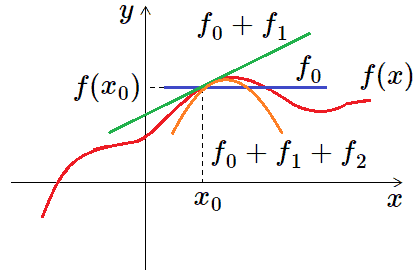As we can see, it touches $f(x)$ better than previous straight line. In the same manner we can proceed and construct approximation that would resemble function $f(x)$ along with derivatives of any order in the vicinity of $x_0$. We’d obtain series of polynomial functions $(x-x_0)^n$. The more terms we add the better approximation we get.

Let function $f(x)$ be differentiable in some vicinity of the point $x=x_0$. Series

$$\sum _{k=0}^{\infty} {\frac {f^{(k)}(x_0 )} {k!} (x-x_0 )^k}=f(x_0 )+\frac{f'(x_0 )}{1!} (x-x_0 )+\frac{f^{\prime \prime} (x_0 )}{2!} (x-x_0 )^2+⋯$$

approximates function $f(x)$ in the vicinity of the point $x_0$. This series is called Taylor series of the function $f(x)$ at the point $x-0$.

In case $x_0=0$, the series is written as follows:

$$\sum _{k=0}^{\infty} {\frac {f^{(k)}(0)} {k!}x^k}=f(0)+\frac{f'(0)}{1!}x+\frac{f^{\prime \prime} (0)}{2!}x^2+⋯$$

This series is called MacLaurin series.Not for any function Taylor series converges. The idea is that in certain cases, not all the time, you can rewrite your function as an infinite sum of other functions. And you only do it in a certain tiny neighborhood of the fixed point of your choice. The following theorem takes place.

Let function $f(x)$ have $n+1$ derivative at some vicinity of the point $x=x_0$. Then, if function can be expanded into series due to powers of $(x-x_0)$ , this expansion is unique and is expressed by the following formula:

\begin{aligned}f(x)=f(x_0 )+\frac{f'(x_0 )}{1!} (x-x_0 )+\frac{f^{\prime \prime} (x_0 )}{2!} (x-x_0 )^2+⋯+R_{n+1}(x)=&\\ \sum _{k=0}^{n} {\frac {f^{(k)}(x_0 )} {k!} (x-x_0 )^k}+…+R_{n+1}(x)\end{aligned}

where $R_{n+1}(x)$ is a remainder term. It can be represented in different ways. Remainder term should be placed if we consider finite number of terms, because in such case our function is approximated only due to certain degree of derivative and no further. This means that approximation and function itself differ and therefrom this remainder term appears. Remainder term, thus, indicates difference between function and its approximation by Taylor series.

But what about $\sin{138}$ we started with, you may ask. In the next section we’ll show you how to obtain Taylor series for common functions and explain how to apply it further in homework tasks. Do math!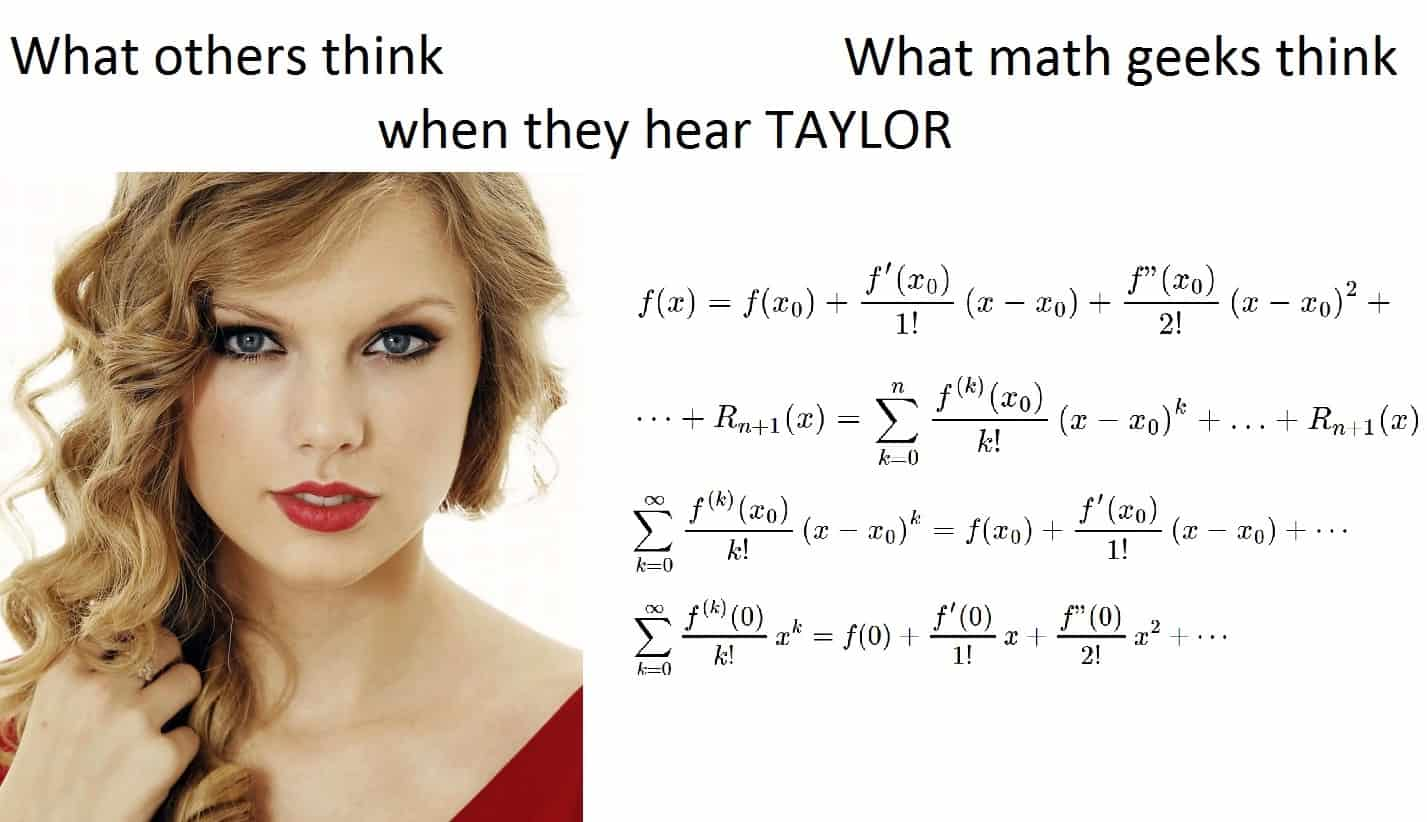0 Shares
Filed under Math.